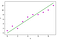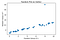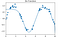A normal or an average person would think that for a complex task to be get executed, it would require matched complex executor. But this assumption doesn’t hold ground, a complex task can be executed by a simple executor. Same is the case with Linear Regression. When I started learning about it, I asked this question (which I assumed that pops up in everyone’s mind). So let’s dive in.

1. Everyone says that Linear Regression should be the first algorithm, begin with, why so?

(Note: If you are here, you might already know that Linear Regression is a Supervised Learning algorithm which needs past data in forms of Label which is nothing but a target variable)

Although a traditional subject in classical statistics, you can also consider Regression from a machine learning point of view. Wikipedia explains it as, “In statistics, linear regression is a linear approach to modelling the relationship between a scalar response or dependent variable and one or more explanatory variables or independent variables” (which is nothing more than a technical jargon ). In more simple words we can say that based on past data or result, we can forecast or predict future data or a result. It is true that this algorithm is quite simple & kinda basic but very powerful (when all assumption holds true or near to true which we’ll later). So trust ‘The Expert’ and start your ML journey with this algorithm.

2. Is it true that a single straight line is able to predict or forecast the data?

First thing first you must know this three concept

Independent variable: They are the features of your target variable and more commonly referred to as just ‘features’ (A simple hack to locate them is just remove the column of target variable and remaining everything from the data set are the features)

Dependent variable: It is the target variable whose values are to be predicted based on features. (A simple hack to locate it is to recognize the column which holds the result from your dataset).

Predictor: It is like the heart and soul of Linear regression. There are factors that influence the outcome of the variable of our interest. There are factors that influence the outcome of the variable of our interest. (Or in more simple words I can put this as the straight line which used in Simple Linear Regression is the predictor).

Now let’s understand how actually is works,

Mathematically, a linear regression is defined by this equation:

y = β0+ β1+ ε

where:

· x is an independent variable.

· y is a dependent variable.

· a is the Y-intercept, which is the expected mean value of y when all x variables are equal to 0.

· b is the slope of a regression line, which is the rate of change for y as x

· ε is the random error term, which is the difference between the actual value of a dependent variable and its predicted value.

-The linear regression equation always has an error term because, in real life, predictors are never perfectly precise.

So the ‘Start’ button for running this model is plot draw a Best fit line. This is achieved by the function defined as:Regression line or Best fit line

Ordinary least squared fit

The farther the points, more the distance and more the cost!

Since this Cost function captures square of the distance, it is known as the least-squares cost function. The idea is to minimize the cost function to get the best fitting line. Linear regression using least squared cost function is known as Ordinary Least Squared Linear Regression (Note: OLS is most widely used, but there are other methods too, to calculate cost function).

For all this technical jargon the simplest form of its explanation would be, after plotting the best fit line, all the value are forecasted by projecting the value of the independent variable on line and then checking respective projected dependent variable’s value.

But more often than not, in real problems, we utilize 2 or more predictors. Such a regression is called Multivariate Regression. Mathematically…

y = b0 x + b1x + …bnxn + a + ε

where:

bn are all the features.

The algorithm works exactly the same for Multivariate Regression like Simple linear regression.

3. Are there any criteria, assumption, etc kind of thing to apply it?

There are some key assumptions that are made whilst dealing with Linear Regression.

These are pretty intuitive and very essential to understand as they play an important role in finding out some relationships in our dataset too!

Let’s discuss these assumptions, their importance and mainly how we validate these assumptions.

i. Linear Relationship Assumption:

Relationship between response (Dependent Variables) and feature variables (Independent Variables) should be linear.

Why it is important:

Linear regression only captures the linear relationship, as it’s trying to fit a linear model to the data

How do we validate it:

The linearity assumption can be tested using scatter plots.

ii. Little or No Multicollinearity Assumption:

It is assumed that there is little or no multicollinearity in the data.

Why it is important:

It results in unstable parameter estimates which makes it very difficult to assess the effect of independent variables.

How to validate it:

Multicollinearity occurs when the features (or independent variables) are not independent from each other.

Pair plots of features help validate

iii. Homoscedasticity Assumption:

Homoscedasticity describes a situation in which the error term (that is, the “noise” or random disturbance in the relationship between the independent variables and the dependent variable) is the same across all values of the independent variables.

Why it is important:

Generally, non-constant variance arises in presence of outliers or extreme leverage values.

How to validate:

A plot between dependent variable vs error.

iv. Little or No autocorrelation in residuals(don’t know…don’t worry):

There should be little or no autocorrelation in the data. Autocorrelation occurs when the residual errors are not independent of each other.

Why it is important:

The presence of correlation in error terms drastically reduces model’s accuracy. This usually occurs in time series models. If the error terms are correlated, the estimated standard errors tend to underestimate the true standard error.

How to validate:

Residual vs time plot. look for the seasonal or correlated pattern in residual values.

v. Normal Distribution of error terms

Why it is important:

Due to the Central Limit Theorem, we may assume that there are lots of underlying facts affecting the process and the sum of these individual errors will tend to behave like in a zero mean normal distribution. In practice, it seems to be so

How to validate:

You can look at QQ plot

4. Ok, it seems that I can work with it, but how does it perform with errors, outliers, etc and how to manage them?

To answer this question first, we must know…

i) Residuals: The difference between the observed value of the dependent variable (y) and the predicted value (ŷ) is called the residual (e). Each data point has one residual.

ii) Outliers: The data point far away from the ‘model’ is basically an Outlier.Example of an outlier( Odd man out)

iii) Correlations: Correlation is a statistical technique that can show whether and how strongly pairs of variables are related. (Or more intuitively calculated by a formula, it ranges from 0 to 1. 0 for not correlated at all and 1 for highly correlated)

If there is one algorithm which is most affected by outliers then it must be the Linear algorithm. Trust me, outliers are one of the major reason behind why most Data Scientists choose to overlook it. Similarly, a high correlation between features is also not healthy for our model.

So here are some useful tips:

i) Remove extreme outliers (this may need your domain knowledge or a human touch, b’coz extreme outliers may be very imp. for the dataset in the real world). Take a log of all feature. Or use Scaling of data (Normalization or standardization)

ii) In Multivariate features, multi-correlation is not a good thing. So pick any one features out of a group of features having a correlation.

5. Polynomial regression looks amazing in the case of multivariate features, so when to use it or actually why not always use it?

Polynomial regression is nothing but taking the n-th degree of your variable. Below is an easy example to display what it does

Say we wanted to take the 1st, 2nd and 3rd degree of the numbers 2,3 & 4.Polynomial regression fitted on Sin Function

Intuitively we know that for 2 the 1st, 2nd and 3rd degrees are: 2(2¹),4(2²) and 8 (2³) (more technical jargon…look at this graph and surely you will understand)

After looking at the graph the first question which pops up is Why not use it always? (at least it happened with me). So we can’t, due to overfitting of data. So let’s understand why so…

Underfitting is when the model fails to capture the overall ‘trend’ of the data. A model that underfits is said to have a high bias.

Overfitting occurs when your model follows the training dataset very rigorously i.e low training error, but it may not work well on generalized or test dataset i.e. high generalization error. An overfitting model is said to have high variance.

To better understand this here is a more general exam, during study a person (say p) study everything by thoroughly mugging from the syllabus and another person (say q) studies by understanding a concept or generalising it. So p will perform well if everything comes from strictly from the syllabus which is not the case in ML. As ML is nothing but generalising our data. So we have to find a Sweet point. Here comes the ultra-important Regularization.

6. Till here it was kind of Ok, but why do we need Regulation? Is it really Important?

Let us understand this by example…

As a parent, Jay said that he is very cautious about the future of his children. He wants them to be successful in life without being strict with them. He took a decision about how much flexibility should be given to his children during their upbringing. Too much restriction may suppress their development of character

Alternatively

Too much flexibility may spoil them. Jay decided to overcome this situation with the idea of regularized(regularization) flexibility, which is to give enough flexibility added with regularization.

By fulfilling some of the expectations of his kids,

like

comic books, drawing setups, storytelling, chocolate, ice cream, mobile game etc. to make them happy.

But added some regularization like:

You have to finish your homework as well; “Distribute chocolate equally with your sister”; Checks exam tests, curfew, etc.

This is an example of a real-life situation, just to build some intuition!

The coefficients of adjacent basis functions grow large and cancel each other out. We need to limit such spikes explicitly in the model by penalizing large values of the model parameters (the thetas of variables)

Such a penalty is known as regularization with two most used types of regularization. It can be done in three ways -

L1 Regularization (also called as Lasso Penalization/Regression)

L2 Regularization (also called as Ridge Penalization/Regression)

Elastic-net

Now follow this two source to understand Regularization thoroughly (this is highly recommended).

Source 1, Source 2(a, b, c)

Note: Do not skip this. Also, follow this channel on youtube- StatQuest By Josh Starmer

7. What is the general blueprint to follow?

acsv → panda dataframe → separate predictors and target variable ‘X,Y’ → split into train & test as 90:10 or 80:20 respectively → model hyper-parameter tunning → split X_test & Y_test into X_test’ and Y_test’ → k-fold → come back to x_train and y_train & fit the data → test it to x_test, y_test → send to mean evaluation r² method

8. That was a lot to take in. Are there any last minute tips and trick or warning?

i) Your data must be linear

ii) Check for outliers.

iii) Somehow if you get an accuracy of 100% over training of data (it may happen, believe me) then you have highly overfitted your data. Throw your model and start again.

iv) You must understand Bias & Variance trade-off. Here is an excellent source/video. See it, do not skip it.

v) Finally practise it again and again, no one understands it in the first time.

Written by

Akash Desarda

GreyAtom is committed to building an educational ecosystem for learners to upskill & help them make a career in data science.

Welcome to a place where words matter. On Medium, smart voices and original ideas take center stage - with no ads in sight. Watch
Follow all the topics you care about, and we’ll deliver the best stories for you to your homepage and inbox. Explore
Get unlimited access to the best stories on Medium — and support writers while you’re at it. Just \$5/month. Upgrade